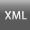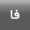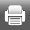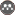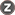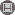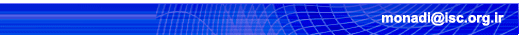[Home ] [Archive]   [ فارسی ]Volume 4, Issue 2 (3-2016)3 2016, 4(2): 3-11 Back to browse issues page
Hyper Elliptic Curve In Cryptography
Abstract:   (3958 Views)

In public key cryptography, systems based on integer factorization problem are increasing replaced by systems based on discrete logarithm problem (DLP). In fact, Elliptic curve cryptography(ECC) makes the key size much smaller than similar RSA systems do that is why ECC became very popular among cryptography system designers. The designers always need to get to a cryptography system with the smallest key size the highest security. Thus they tend to use hyper elliptic curve in cryptography. In this paper, we will study how to use this type of curves in cryptography.

Also, this study takes a look at these curves’ resistance against algorithms of solving the discrete logarithm problem. Energy consumed for implementation of the scalar multiplication in hyper elliptic curves of the genus g<5 will be analyzed too.

Keywords: Cryptography, discrete logarithm problem, hyperelliptic curve, scalar multiplication.‎
Type of Study: Scientific extension | Subject: Special
Received: 2015/12/30 | Accepted: 2016/11/21 | Published: 2016/11/21
Send email to the article author